# 10.8 Vectors  (Page 7/22)

 Page 7 / 22

## Verbal

What are the characteristics of the letters that are commonly used to represent vectors?

lowercase, bold letter, usually $\text{\hspace{0.17em}}u,v,w$

How is a vector more specific than a line segment?

What are $\text{\hspace{0.17em}}i\text{\hspace{0.17em}}$ and $\text{\hspace{0.17em}}j,$ and what do they represent?

They are unit vectors. They are used to represent the horizontal and vertical components of a vector. They each have a magnitude of 1.

What is component form?

When a unit vector is expressed as $⟨a,b⟩,$ which letter is the coefficient of the $\text{\hspace{0.17em}}i\text{\hspace{0.17em}}$ and which the $\text{\hspace{0.17em}}j?$

The first number always represents the coefficient of the $\text{\hspace{0.17em}}i,\text{\hspace{0.17em}}$ and the second represents the $\text{\hspace{0.17em}}j.$

## Algebraic

Given a vector with initial point $\text{\hspace{0.17em}}\left(5,2\right)\text{\hspace{0.17em}}$ and terminal point $\text{\hspace{0.17em}}\left(-1,-3\right),\text{\hspace{0.17em}}$ find an equivalent vector whose initial point is $\text{\hspace{0.17em}}\left(0,0\right).\text{\hspace{0.17em}}$ Write the vector in component form $⟨a,b⟩.$

Given a vector with initial point $\text{\hspace{0.17em}}\left(-4,2\right)\text{\hspace{0.17em}}$ and terminal point $\text{\hspace{0.17em}}\left(3,-3\right),\text{\hspace{0.17em}}$ find an equivalent vector whose initial point is $\text{\hspace{0.17em}}\left(0,0\right).\text{\hspace{0.17em}}$ Write the vector in component form $⟨a,b⟩.$

$〈7,-5〉$

Given a vector with initial point $\text{\hspace{0.17em}}\left(7,-1\right)\text{\hspace{0.17em}}$ and terminal point $\text{\hspace{0.17em}}\left(-1,-7\right),\text{\hspace{0.17em}}$ find an equivalent vector whose initial point is $\text{\hspace{0.17em}}\left(0,0\right).\text{\hspace{0.17em}}$ Write the vector in component form $⟨a,b⟩.$

For the following exercises, determine whether the two vectors $\text{\hspace{0.17em}}u\text{\hspace{0.17em}}$ and $\text{\hspace{0.17em}}v\text{\hspace{0.17em}}$ are equal, where $\text{\hspace{0.17em}}u\text{\hspace{0.17em}}$ has an initial point $\text{\hspace{0.17em}}{P}_{1}\text{\hspace{0.17em}}$ and a terminal point $\text{\hspace{0.17em}}{P}_{2}\text{\hspace{0.17em}}$ and $v$ has an initial point $\text{\hspace{0.17em}}{P}_{3}\text{\hspace{0.17em}}$ and a terminal point $\text{\hspace{0.17em}}{P}_{4}$ .

${P}_{1}=\left(5,1\right),{P}_{2}=\left(3,-2\right),{P}_{3}=\left(-1,3\right),\text{\hspace{0.17em}}$ and $\text{\hspace{0.17em}}{P}_{4}=\left(9,-4\right)$

not equal

${P}_{1}=\left(2,-3\right),{P}_{2}=\left(5,1\right),{P}_{3}=\left(6,-1\right),\text{\hspace{0.17em}}$ and $\text{\hspace{0.17em}}{P}_{4}=\left(9,3\right)$

${P}_{1}=\left(-1,-1\right),{P}_{2}=\left(-4,5\right),{P}_{3}=\left(-10,6\right),\text{\hspace{0.17em}}$ and $\text{\hspace{0.17em}}{P}_{4}=\left(-13,12\right)$

equal

${P}_{1}=\left(3,7\right),{P}_{2}=\left(2,1\right),{P}_{3}=\left(1,2\right),\text{\hspace{0.17em}}$ and $\text{\hspace{0.17em}}{P}_{4}=\left(-1,-4\right)$

${P}_{1}=\left(8,3\right),{P}_{2}=\left(6,5\right),{P}_{3}=\left(11,8\right),\text{\hspace{0.17em}}$ and ${P}_{4}=\left(9,10\right)$

equal

Given initial point $\text{\hspace{0.17em}}{P}_{1}=\left(-3,1\right)\text{\hspace{0.17em}}$ and terminal point $\text{\hspace{0.17em}}{P}_{2}=\left(5,2\right),\text{\hspace{0.17em}}$ write the vector $\text{\hspace{0.17em}}v\text{\hspace{0.17em}}$ in terms of $\text{\hspace{0.17em}}i\text{\hspace{0.17em}}$ and $\text{\hspace{0.17em}}j.\text{\hspace{0.17em}}$

Given initial point $\text{\hspace{0.17em}}{P}_{1}=\left(6,0\right)\text{\hspace{0.17em}}$ and terminal point $\text{\hspace{0.17em}}{P}_{2}=\left(-1,-3\right),\text{\hspace{0.17em}}$ write the vector $\text{\hspace{0.17em}}v\text{\hspace{0.17em}}$ in terms of $\text{\hspace{0.17em}}i\text{\hspace{0.17em}}$ and $\text{\hspace{0.17em}}j.\text{\hspace{0.17em}}$

$7i-3j$

For the following exercises, use the vectors u = i + 5 j , v = −2 i − 3 j ,  and w = 4 i j .

Find u + ( v w )

Find 4 v + 2 u

$-6i-2j$

For the following exercises, use the given vectors to compute u + v , u v , and 2 u − 3 v .

$u=⟨2,-3⟩,v=⟨1,5⟩$

$u=⟨-3,4⟩,v=⟨-2,1⟩$

$u+v=〈-5,5〉,u-v=〈-1,3〉,2u-3v=〈0,5〉$

Let v = −4 i + 3 j . Find a vector that is half the length and points in the same direction as $\text{\hspace{0.17em}}v.$

Let v = 5 i + 2 j . Find a vector that is twice the length and points in the opposite direction as $\text{\hspace{0.17em}}v.$

$-10i–4j$

For the following exercises, find a unit vector in the same direction as the given vector.

a = 3 i + 4 j

b = −2 i + 5 j

$-\frac{2\sqrt{29}}{29}i+\frac{5\sqrt{29}}{29}j$

c = 10 i j

$d=-\frac{1}{3}i+\frac{5}{2}j$

$-\frac{2\sqrt{229}}{229}i+\frac{15\sqrt{229}}{229}j$

u = 100 i + 200 j

u = −14 i + 2 j

$-\frac{7\sqrt{2}}{10}i+\frac{\sqrt{2}}{10}j$

For the following exercises, find the magnitude and direction of the vector, $\text{\hspace{0.17em}}0\le \theta <2\pi .$

$⟨0,4⟩$

$⟨6,5⟩$

$|v|=7.810,\theta =39.806°$

$⟨2,-5⟩$

$⟨-4,-6⟩$

$|v|=7.211,\theta =236.310°$

Given u = 3 i − 4 j and v = −2 i + 3 j , calculate $\text{\hspace{0.17em}}u\cdot v.$

Given u = − i j and v = i + 5 j , calculate $\text{\hspace{0.17em}}u\cdot v.$

$-6$

Given $\text{\hspace{0.17em}}u=⟨-2,4⟩\text{\hspace{0.17em}}$ and $\text{\hspace{0.17em}}v=⟨-3,1⟩,\text{\hspace{0.17em}}$ calculate $\text{\hspace{0.17em}}u\cdot v.$

Given u $=⟨-1,6⟩$ and v $=⟨6,-1⟩,$ calculate $\text{\hspace{0.17em}}u\cdot v.$

$-12$

## Graphical

For the following exercises, given $\text{\hspace{0.17em}}v,\text{\hspace{0.17em}}$ draw $v,$ 3 v and $\text{\hspace{0.17em}}\frac{1}{2}v.$

$⟨2,-1⟩$

$⟨-1,4⟩$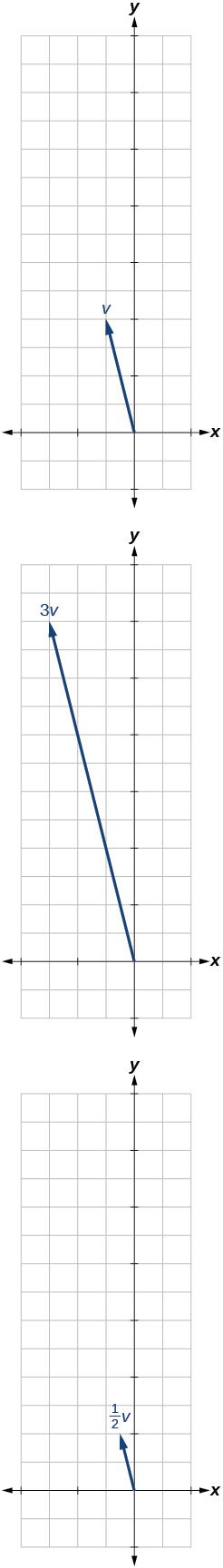$⟨-3,-2⟩$

For the following exercises, use the vectors shown to sketch u + v , u v , and 2 u .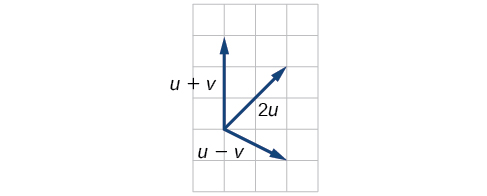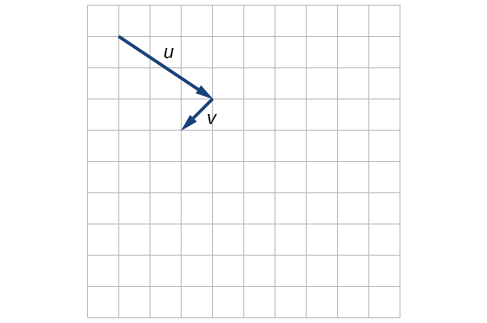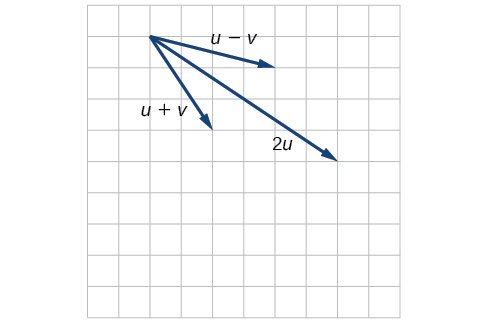For the following exercises, use the vectors shown to sketch 2 u + v .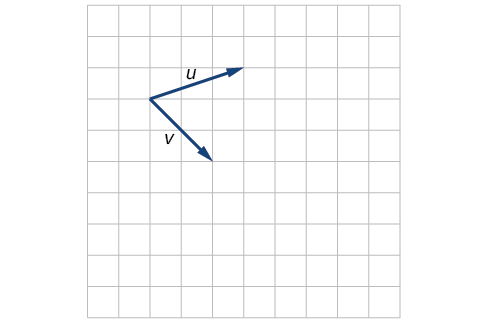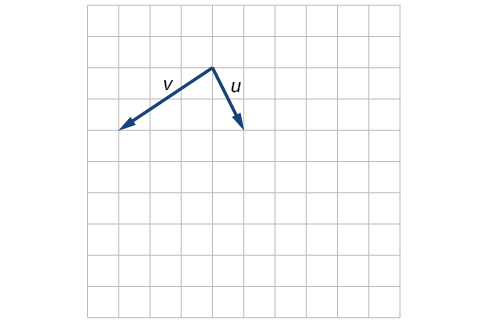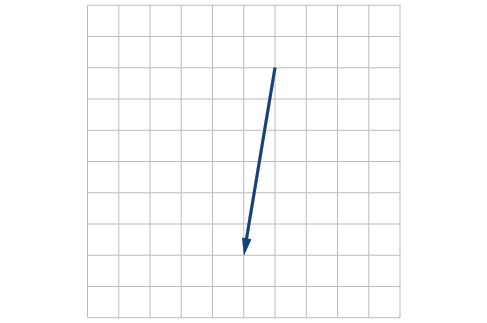For the following exercises, use the vectors shown to sketch u − 3 v .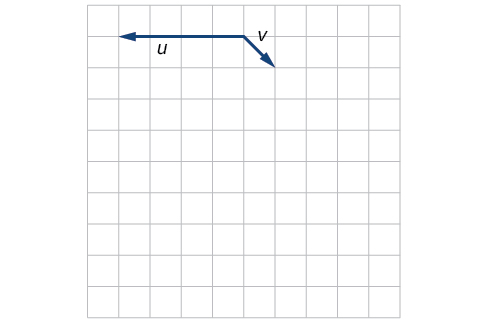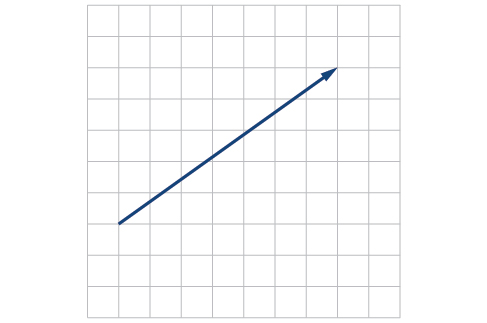For the following exercises, write the vector shown in component form.

stock therom F=(x2+y2) i-2xy J jaha x=a y=o y=b
root under 3-root under 2 by 5 y square
The sum of the first n terms of a certain series is 2^n-1, Show that , this series is Geometric and Find the formula of the n^th
cosA\1+sinA=secA-tanA
why two x + seven is equal to nineteen.
The numbers cannot be combined with the x
Othman
2x + 7 =19
humberto
2x +7=19. 2x=19 - 7 2x=12 x=6
Yvonne
because x is 6
SAIDI
what is the best practice that will address the issue on this topic? anyone who can help me. i'm working on my action research.
simplify each radical by removing as many factors as possible (a) √75
how is infinity bidder from undefined?
what is the value of x in 4x-2+3
give the complete question
Shanky
4x=3-2 4x=1 x=1+4 x=5 5x
Olaiya
hi can you give another equation I'd like to solve it
Daniel
what is the value of x in 4x-2+3
Olaiya
if 4x-2+3 = 0 then 4x = 2-3 4x = -1 x = -(1÷4) is the answer.
Jacob
4x-2+3 4x=-3+2 4×=-1 4×/4=-1/4
LUTHO
then x=-1/4
LUTHO
4x-2+3 4x=-3+2 4x=-1 4x÷4=-1÷4 x=-1÷4
LUTHO
A research student is working with a culture of bacteria that doubles in size every twenty minutes. The initial population count was  1350  bacteria. Rounding to five significant digits, write an exponential equation representing this situation. To the nearest whole number, what is the population size after  3  hours?
v=lbh calculate the volume if i.l=5cm, b=2cm ,h=3cm
Need help with math
Peya
can you help me on this topic of Geometry if l help you
litshani
( cosec Q _ cot Q ) whole spuare = 1_cosQ / 1+cosQ
A guy wire for a suspension bridge runs from the ground diagonally to the top of the closest pylon to make a triangle. We can use the Pythagorean Theorem to find the length of guy wire needed. The square of the distance between the wire on the ground and the pylon on the ground is 90,000 feet. The square of the height of the pylon is 160,000 feet. So, the length of the guy wire can be found by evaluating √(90000+160000). What is the length of the guy wire?
the indicated sum of a sequence is known as
how do I attempted a trig number as a starterByByBy Briana KnowltonBy OpenStaxBy Jonathan LongBy OpenStaxBy Jesenia WoffordBy Yasser IbrahimBy Katy PrattBy Joanna SmithbackBy Anonymous UserBy Madison Christian